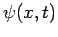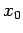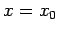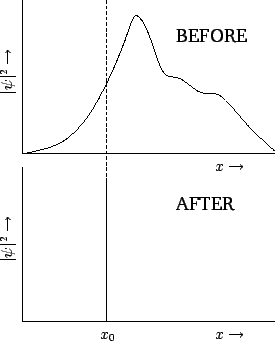Next: Exercises Up: Wave-Particle Duality Previous: Schrödinger's Equation

# Collapse of the Wave Function

Consider an extended wavefunction. According to our usual interpretation,is proportional to the probability density of a measurement of the particle's position yielding the valueat time. If the wavefunction is extended then there is a wide range of likely values that this measurement could give. Suppose that we make such a measurement, and obtain the value. We now know that the particle is located at. If we make another measurement immediately after the first one then what value do we expect to obtain? Well, common sense tells us that we must obtain the same value,, since the particle cannot have shifted position appreciably in an infinitesimal time interval. Thus, immediately after the first measurement, a measurement of the particle's position is certain to give the value, and has no chance of giving any other value. This implies that the wavefunction must have collapsed to some sort of spike'' function located at. This is illustrated in Fig. 9. Of course, as soon as the wavefunction has collapsed, it starts to expand again, as discussed in Sect. 3.13. Thus, the second measurement must be made reasonably quickly after the first, in order to guarantee that the same result will be obtained.The above discussion illustrates an important point in quantum mechanics. Namely, that the wavefunction of a particle changes discontinuously (in time) whenever a measurement is made. We conclude that there are two types of time evolution of the wavefunction in quantum mechanics. First, there is a smooth evolution which is governed by Schrödinger's equation. This evolution takes place between measurements. Second, there is a discontinuous evolution which takes place each time a measurement is made.

SubsectionsNext: Exercises Up: Wave-Particle Duality Previous: Schrödinger's Equation
Richard Fitzpatrick 2010-07-20